• c

posted on 23 Oct 2020 under category note

• 不要迷信书中某些术语, 比如 “有名常量”… 可能叫法和惯例不同
• 书中内容及惯例用法可能有些过时, 一定要买其他书参考进阶. 推荐 <K&R> 以及 <C 语言: 现代方法>
• 教材多为一些知识点的罗列, 这这片文章更是对罗列点的罗列
• 教材侧重对高级语言程序设计概念的讲解, 绝不是针对 C 语言学习的首选参考物

# 基础

C 中允许出现的所有基本字符的组合称为 C 语言的字符集, 包括:

• A~Z, a~z (52 个)
• 数字 (10 个)
• 其他可显示字符 (33 个)
• 转义字符

``````~ ` ! @ # \$ % ^ & * ( ) _ - + = | \ { } [ ] : ; " ' < > , . ? / 空格
``````

``````\n \t \v \b \r \f \a \" \' \\ \ddd \xhh
``````

``````auto break case char continue const default do double else enum
extern float for goto int if long register return short signed
sizeof static struct switch typedef union unsigned void volatile while
``````

• `/* */`
• `//`

# 数据类型, 运算符和表达式

## 常量 (字面量)

### 整形

• 十进制整数: `0`, `-111`
• 八进制整数, 以 0 开头: `00`, `-0111`
• 十六进制整数, 以 0x 开头: `0x0`, `-0x111`

• 短整型: 一般占 2 字节, 范围 -32768~+32767
• 长整形: 占 4 字节, 范围 -2147483648~+2147483647, 末尾加 l 或 L, 如 `100l`. 如果没有加 l 但超出短整型范围, 则自动认为该常量是长整形

### 实型

• 一般形式: `12.345`, `-.234`, `47.`. 注意小数点前后数字可省略
• 指数形式: `12.345e-2`, `-1.2345E3`

### 字符

• 使用单引号括住的单个字符, 如 `'a'`, `'1'`
• 也可以使用转义字符如 `'\n'`, `'\101'` (8进制), `'\x41'` (16进制)

### 符号常量

• 符号常量名建议用大写字母
• 一般放在程序开头, 每个定义独占一行
• 后面不用跟分号

`#define` 是一条宏定义命令.

``````#define R1 2+3
2 * R1; // 将被替换为 2 * 2+3, 这可能不是你想要的!
``````

## 变量

• 变量名命名规则同标识符
• 定义变量后, 会自动分配连续的内存单元
• 可用的_基本_数据类型符见后表

## 运算符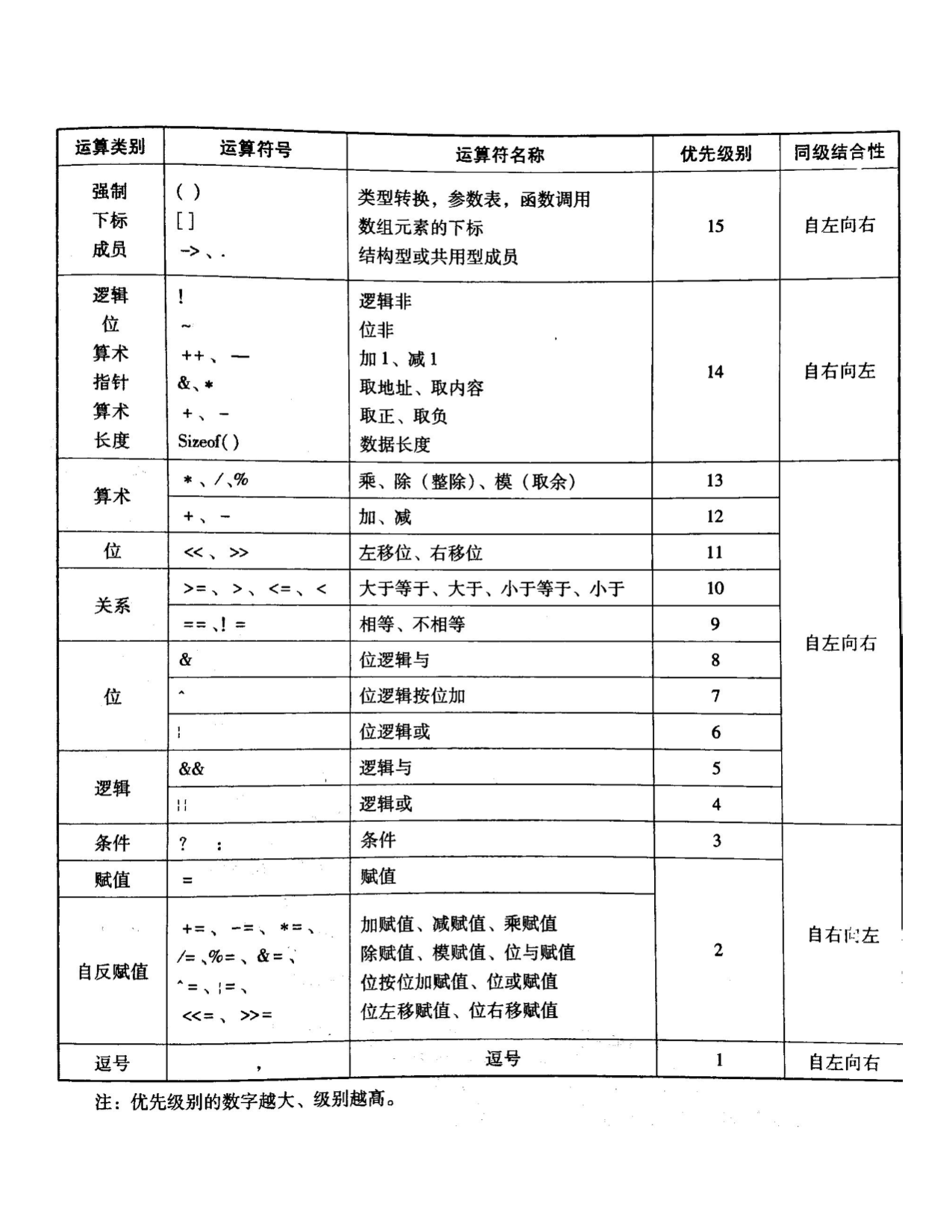• 模运算的对象必须是整型数据, 且结果的符号必须与被除数相同.
• 可以使用圆括号来表示运算的顺序, 增加程序的可读性.
• `&&``||` 会造成短路运算.
• 逗号运算符 `,` 是双目运算符, 运算对象是表达式, 运算结果值为 最右边表达式的值
• `sizeof` 的运算对象可以是数据类型符 (`sizeof int`), 也可以是变量 (`sizeof a`)
• 移位运算符: 左移时补 0, 右移时, 如果是带符号数则补符号位, 否则补 0. 对某个对象移位后, 原对象不变.
``````13 % -3; // 1
3 > 2 > 1; // 0 (假). 相当于 (3 > 2) > 1

int a = 10, b = 10, c = 10, d = 10;
a += b -= c *= d /= 2; // -30. 相当于 a += (b -= (c *= (d /= 2)))

a == b ? (c = 1) : (d = 0); // 由于条件运算符优先于赋值运算符, 圆括号不能少
``````

## 类型转换规则

• 表达式计算时, 就长不就短: 参与运算的数据都转换成数据长度最长的数据类型, 然后计算. 结果值的类型就是数据长度最长的数据类型.
• 运算结果存入变量时: 就左不就右: 先将结果自动转换成左边变量的类型, 然后赋予该变量.
• 强制转换: `(数据类型符) (表达式)`
• 把数据较长的结果存入较短的变量时, 将截去超长的部分, 可能造成数据错误
• 过多的类型转换会降低运行效率

# 控制结构语句

## 顺序结构

• 赋值语句: `变量 = 表达式`
• 函数调用语句: `函数名(参数...)`
• 表达式语句: `表达式;`

任何表达式都能构成语句. 在表达式后面跟一个分号 `;`, 构成的语句就是 表达式语句. 赋值语句, 函数调用语句都是表达式语句的特例

• 复合语句: `{ 语句;... }`. 复合语句中若有数据定义语句, 要放在其他语句前面

## 选择结构

### if … else

``````if (表达式) {
语句;
} else {
语句;
}
``````
• 可以嵌套
• 表达式可以是任何类型, 常用的是关系表达式或逻辑表达式
• `else` 总是和前面最近的 `if` 配对

### switch … case

``````switch (表达式) {
case 常量表达式: 语句组;
break;
...
default: 语句组;
}
``````
• 可以嵌套
• 表达式常用的是字符型或整形表达式
• 常量表达式是由常量或符号常量组成的表达式

## 循环结构

• 语句称为循环体, 可以是任何语句, 通常是复合语句
• 可以嵌套
• 表达式可以是任何类型, 常用关系型或逻辑型表达式

### while

``````while (表达式) {
语句;
}
``````

### do … while

``````do {
语句;
} while (表达式);
``````

### for

``````for (int i = 0; i < 11; i++) {
语句;
}
``````

### break

• 强制结束当前循环
• 只能用在三条循环语句的循环体中或 `switch`

### continue

• 跳过 `continue` 之后的语句, 重新判断循环控制条件决定是否循环
• 只能用在三条循环语句的循环体中

# 数组

## 一维数组

• 下表从 0 开始
• 数组元素要参与表达式运算, 必须已经被赋值
• 引用数组元素时, 系统不检查下标是否越界

• 若所有元素均赋初值, 长度可省略
• 对于没有给出初值的元素, 数值型数组默认初值为 `0`, 字符型数组默认初值为 `'\0'`

## 二维数组

• 分行赋初值: `int a = { {1, 2, 3}, {4, 5, 6} };`
• 不分行赋初值: `int a = {1, 2, 3, 4, 5, 6};`
• 对每行前若干元素赋初值: `int a = { {1}, {4, 5} };`
• 对若干行前若干元素赋初值: `int a = { {1, 2} };`
• 给所有元素赋初值, 行长度可省略: `float a[] = { {1, 2, 3}, {4, 5, 6} };`
• 分行给所有行前若干元素赋初值, 行长度可省略: `float a[] = { {1}, {2, 3} };`

## 字符数组与字符串

• 同普通数组方式
• 直接用字符串常量: 注意数组长度至少要比字符串字符数多 1, 以存放 `\0`
• `char s = {"12"};`
• `char s[] = "string";`

## 输入输出函数

• 输入: `getchar()`. 从键盘读取单个字符
• 输出: `putchar(ch)`. 将 `ch` 对应的字符输出到显示器上

• 输入: `gets(字符数组)`
• 输出: `puts(字符数组)`

• 输入: `scanf(输入格式字符串, 输入变量地址表)`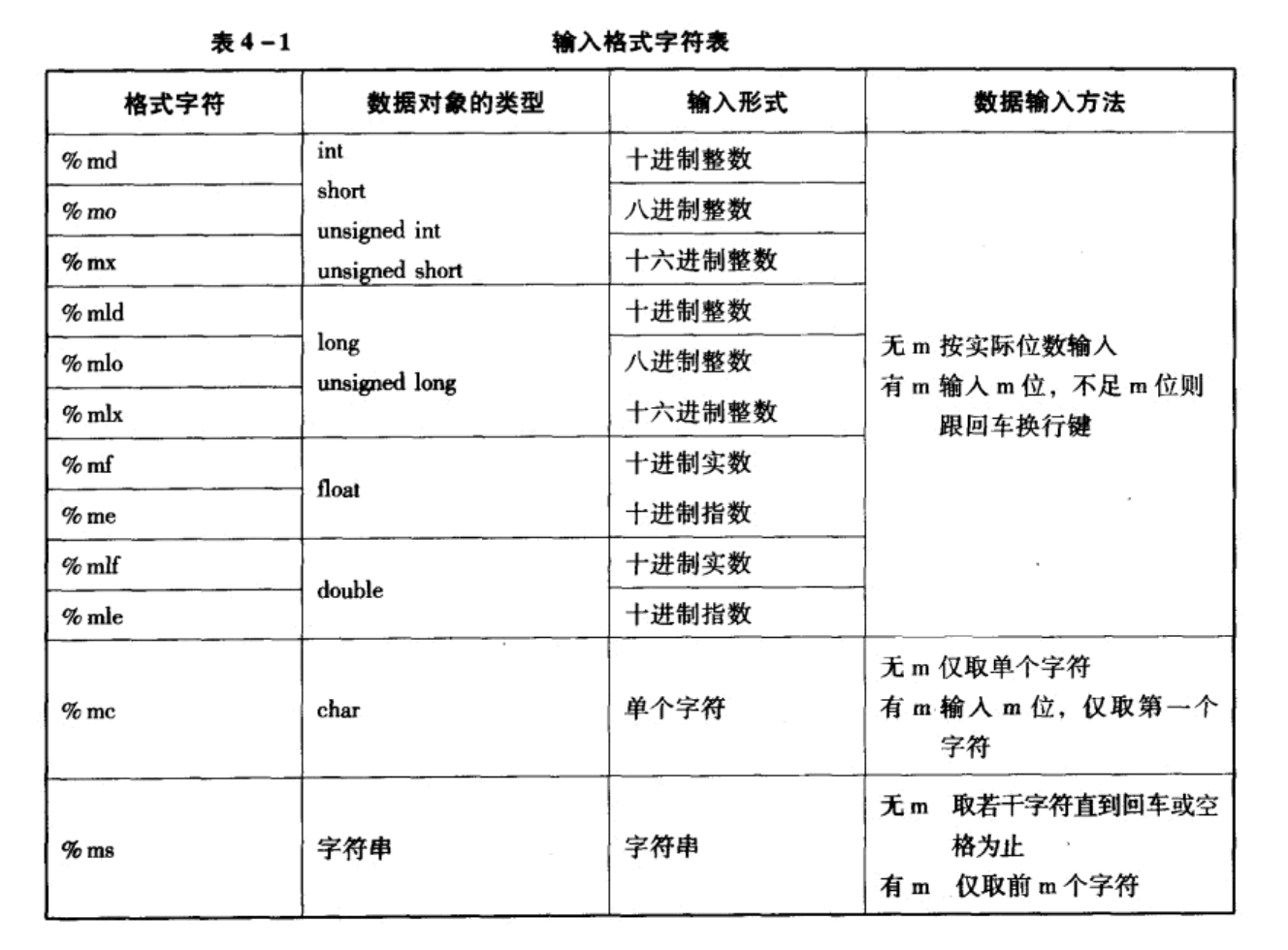• 输出: `printf(输出格式字符串, 输出表达式表)`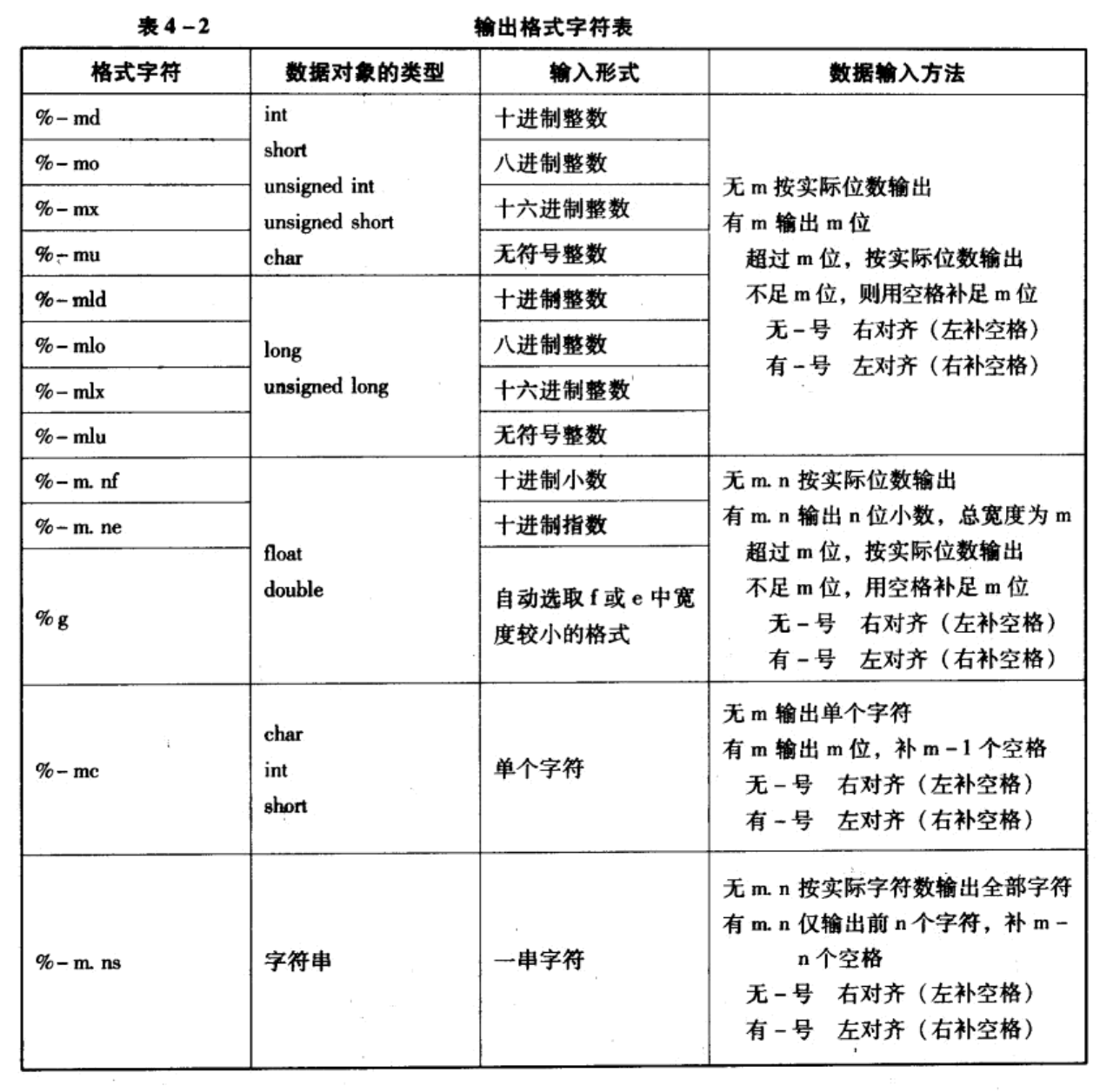``````// 对于 printf(), \0 不输出
// 对于 puts(), \0 输出成回车换行符
puts("string");
printf("%s%s", "string", "string"); // 字符串结束标记符不输出
// 输出:
// string
// stringstring

// 对于 scanf(), 回车换行符或空格符看成输入结束
// 对于 gets(), 只有回车换行符看成输入结束, 空格看做字符串一部分
char a, b;
gets(a);
scanf("%s", b);
// 如果输入:
// 123 xyz
// 345 xyz
// 则 a 为 123 xyz, b 为 345
``````

# 指针

• 对于变量: 通过变量名查取变量地址, 再从变量对应地址的字节中取得值获奖某值存入变量对应地址的字节中
• 对于数组: 通过查数组与地址对照表获得数组首地址, 通过计算元素地址的方式先获得元素地址, 再取值或存值

• 数据类型符指的是要指向的变量或数组的数据类型
• 初值可以是: `&变量名`, `&数组元素`, 一维数组名, 其他指针变量, `0`
• 初值中指定的变量名或数组必须已经定义

• 变量地址只能利用取地址运算符 `&` 来获得
• `&` 的运算对象是变量或数组元素名, 运算结果是其地址
• C 语言规定, 一维数组名可以看成是地址常量, 其值是一维数组的首地址. 而不允许把整数看成地址常量, 所以地址型表达式不能是整数

• 需要事先将指针变量指向这个变量或数组
• `*指针变量名` 则代表它所指向的变量或数组元素. `*` 称为指针运算符
• 当指针变量的值为一维数组的首地址时, 它代表数组的第一个元素
``````float a, *pa = &a;
// *&a 是正确的. 相当于 *(&a), &a 是变量 a 的地址, *(a 的地址) 代表变量 a
// &*a 是错误的. 相当于 &(*a), 因为 a 不是指针变量, 所以 *a 不正确
// *&pa 是正确的. 相当于 *(&pa), &pa 是 pa 的地址, *(pa 的地址) 代表 pa
// &*pa 是正确的. 相当于 &(*pa), *pa 代表变量 a, &(变量 a) 代表 a 的地址

int i, *pi = &i;
// (*pi)++ 小括号必须, 因为 * 和 ++ 由右向左结合
// ++*pi 小括号非必须, 理由同上
``````

## 指向一维数组的指针变量

• 初始化: `*指针变量 = 数组名`
• 赋值: `指针变量 = 数组名`

• 初始化: `*指针变量 = &数组名[下标]`
• 赋值: `指针变量 = &数组名[下标]`

• 指针法: `*(指针变量+i)``*(数组名+i)`
• 下标法: `指针变量[i]``数组名[i]`

虽然从书写格式上看, 指向一维数组的指针变量和数组名可以互换, 但它们是完全不同的概念. 指针变量是存放地址型数据的变量, 可以按照变量的处理方式对其进行运算或赋值; 而数组名代表数组首地址, 是一个地址常量, 只能按常量方式使用. 例如 `pa = a` 是正确的, 因为对变量可以赋值, 而 `a = pa` 是错误的, 因为不能对常量进行赋值.

• `指针变量 + 整数`
• `指针变量 - 整数`
• `++指针变量`
• `--指针变量`
• `指针变量++`
• `指针变量--`

运算结果为: (指针变量中的地址) 和 (整数 * 指针变量类型占用字节数) 运算之后的地址.

上述规则组成的表达式即地址型表达式指针型表达式

``````short a, *pa = a, *pb = a;
pa = pa + 5; // pa 指向了 a
pa--; // pa 指向了 a
pa - pb; // 4
pa < pb; // 假
``````

## 指向字符串常量的指针变量

• 初始化: `*指针变量 = 字符串常量`
• 赋值: `指针变量 = 字符串常量`

注意: 虽然可以用赋值方式使指针变量指向字符串常量, 但不允许将字符串常量用赋值方式赋予字符型数组

``````char *p = "abcd";
char *p;
p = "abcd";

char a;
a = "abcd";
``````

``````// 初始化:
// - 使用数组保存字符串
// 1.
char s = {"string"};
// 2.
char s = "string"
// 3.
char s[] = "string";
// - 使用指针指向字符串
char *ps = "string";

// 赋值:
// - 使用数组: 报错!
s = "another";
// - 指针则可以
ps = "another";

// 如果 ps 指向 s
char s = "string", *ps = s;
ps = "another";
// 注意, s 值并没有改变. 因为只是把 ps 从指向 s 变为指向另一个字符串常量 "another"
// 如果想改变 s 值, 可以使用 strcpy()
strcpy(ps, "another");
// 或
strcpy(s, "another");
``````

## 指针数组

• `指针数组名[下标] + 整数`
• `指针数组名[下标] - 整数`
• `++指针数组名[下标]`
• `--指针数组名[下标]`
• `指针数组名[下标]++`
• `指针数组名[下标]--`

# 函数

## 函数定义

``````存储类型符 数据类型符 函数名(形式参数表) {
数据定义语句序列;
执行语句序列;
}
``````

• 存储类型符可以是 `extern``static`, 也可以省略, 默认 `extern`
• `extern`: 定义外部函数, 它可以被其他编译单位中的函数调用
• `static:` 定义内部函数, 它只能被本编译单位中的函数调用
• 数据类型符可以是各种基本类型符, 指针型 (函数名前加 `*` 即可) 或 `void` (代表无返回值)
• 形式参数表格式: `数据类型符 形式参数, ...`
• 当形式参数是数组时, 一维数组数组的长度, 二维数组的行长度可以省略

## 函数调用

• 书写顺序上, 被调函数在主调函数之前
• 被调函数在之后, 但它有返回值且返回整形或字符型

• 形参是变量时: 使用值传递方式

计算实参的值, 并把值赋值给形参, 然后在函数体内参与运算. 形参的值改变不影响实参.

• 形参是数组时: 使用地址传递方式

实参是地址型的表达式, 例如数组的首地址, 或已经赋值的指针变量, 指针数组元素等. 将实参的 地址 赋予形参数组作为其首地址. 形参数组不分配内存, 占用和实参一样的内存空间. 对形参数组的改变如同对实参的改变.

• 形参是指针变量时: 使用地址值的值传递方式

实参必须是地址表达式. 函数调用时为形参分配内存, 计算实参表达式的地址值赋予形参指针. 在被调函数中, 形参对应的指针变量已经指向主函数的某个变量. 所以使用形参指针可以修改主调函数中的变量或数组元素的值.

## 变量的存储类型和作用域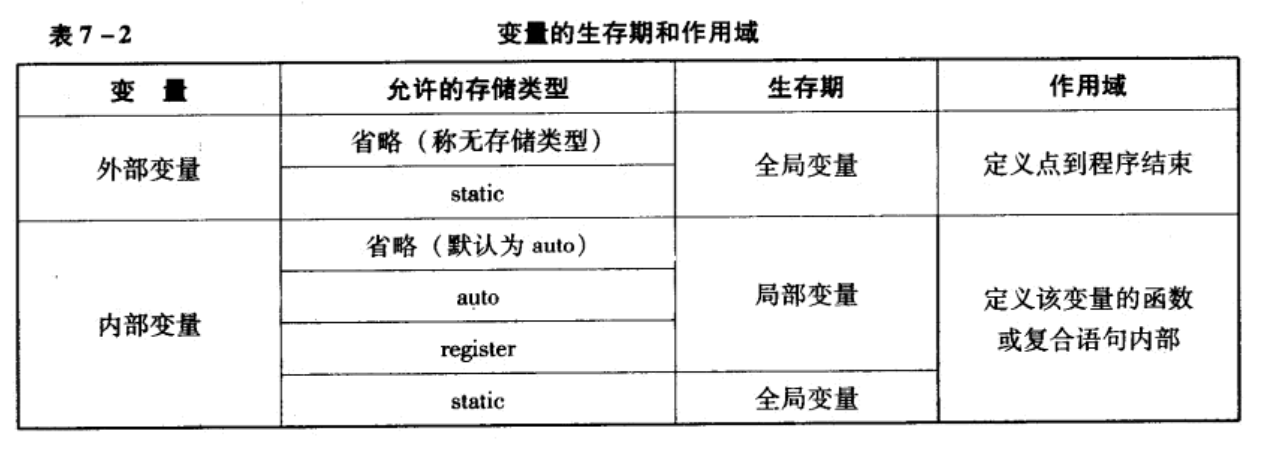• 外部变量不能重名. 如果全局外部变量和内部变量重名, 内部变量优先.
• 不推荐使用全局变量, 它破坏了模块的独立性, 程序可读性差

## 多文件程序的处理

• `#include <文件名>` 系统仅按规定的路径搜索文件
• `#include "文件名"` 先在本程序所在路径下寻找文件, 找不到再在系统规定的路径搜索文件

## 常用系统函数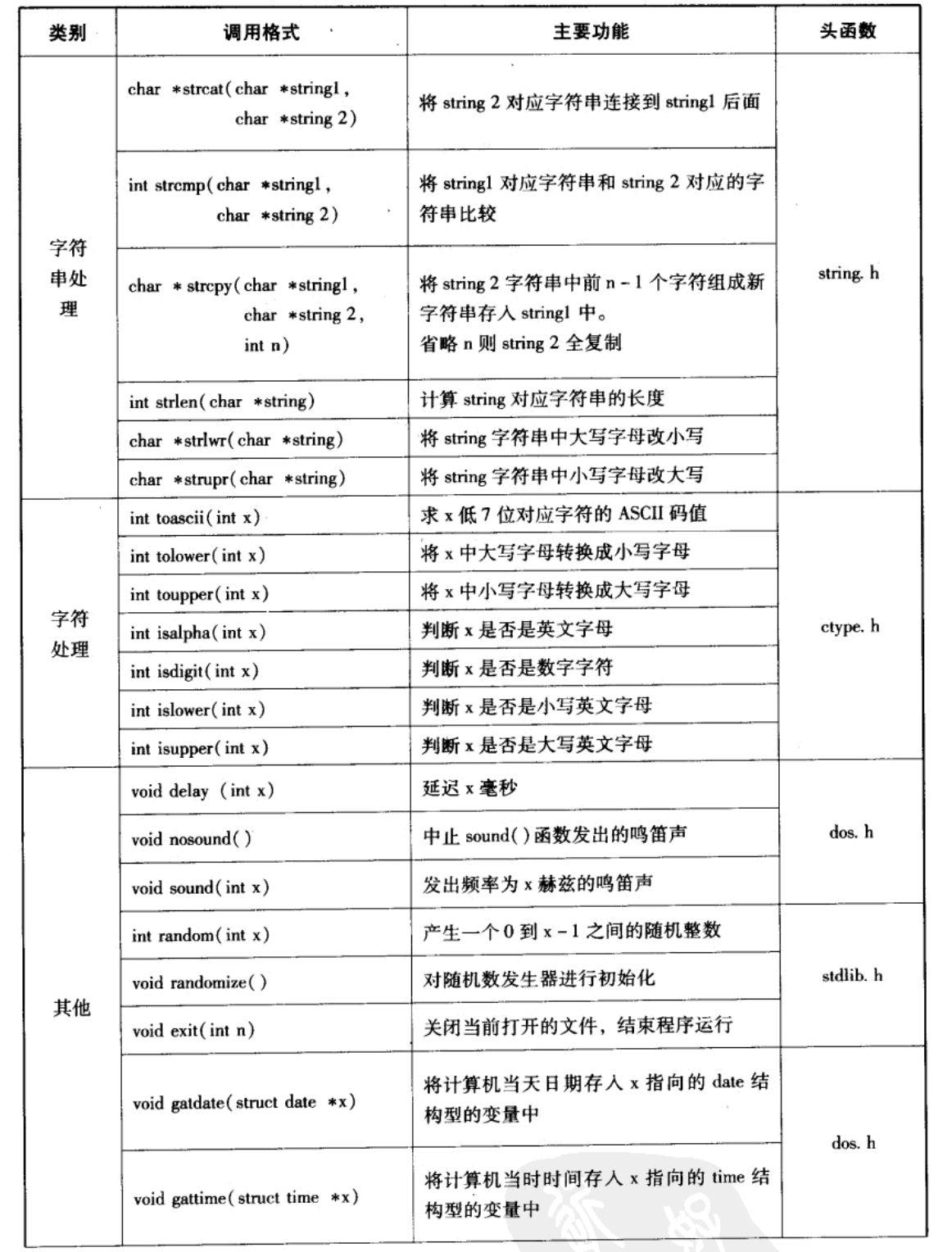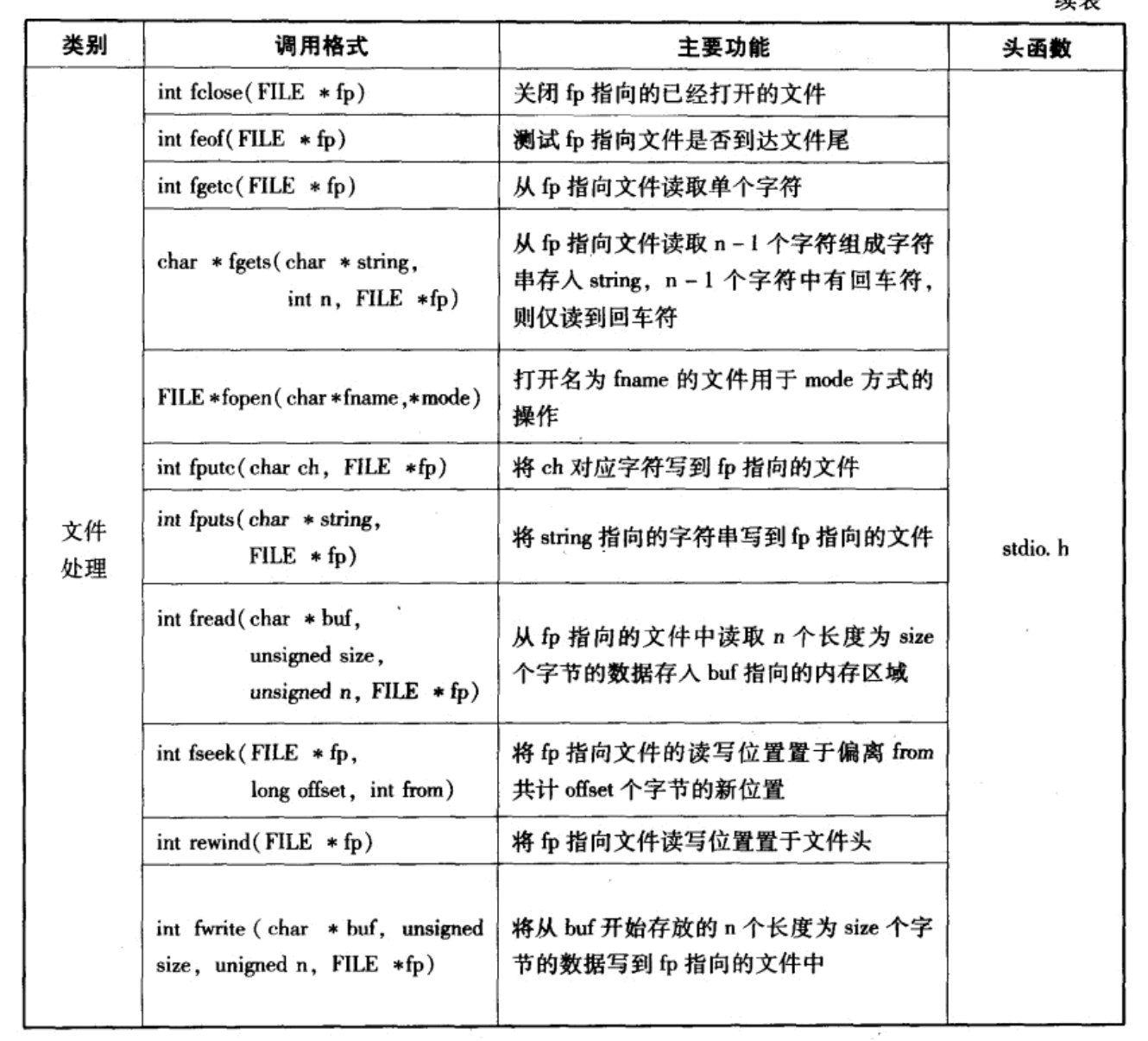# 结构型

``````struct 结构型名 {
数据类型符 成员名;
...
};
``````
• 结构型定义可以嵌套
• 成员也成, 结构分量
• 结构型仅仅是一种数据类型, 系统不会给结构型成员分配内存
``````// 定义结构型
struct birthday {
int year;
int month;
int day;
};

// 定义结构型, 同时定义结构型变量
// 可以省略结构型名称 person, 变成无名称的结构型. 但这样做的话, 以后将无法使用这个结构型定义其他变量
struct person {
char name;
char sex;
struct birthday bir; // 嵌套结构型, 注意前面的 struct
float wage;
} a = {100001l, "zhao", 'f', {89, 94, 86} }, // 结构型变量
b = { {100002l, "qian", 'm', {78, 86, 92} }, // 结构型数组
{100003l, "sun", 'f', {85, 68, 82} } };

// 声明结构型变量
struct person b, c;

// 声明结构型变量, 并赋初值
struct person e = {100001l, "zhao", 'f', {89, 94, 86}},
f = {100002l, "qian", 'm', {78, 86, 92}};

// 声明结构型数组
struct person p = { {100003l, "sun", 'f', {78, 86, 92} },
{100004l, "li", 'm', {85, 69, 92} } };

// 引用结构型变量成员
e.birthday.year;

// 引用成员地址
&e.birthday.year;

// 引用结构型变量地址
&e;

// 引用结构型数组元素成员
p.birthday.year;

// 引用结构型数组元素成员地址
&p.birthday;

// 引用结构型数组元素地址
&p;

// 引用结构型数组首地址
p
``````

• 成员是变量或者数组首地址: `&结构型变量明.成员名`
• 成员是数组, 引用其元素地址: `&结构型变量名.成员数组[下标]`

. 称为成员运算符.

## 指向结构型数据的指针变量

### 指向结构型变量

• (*指针变量).成员名 必须加括号, 因为 . 优先级比 * 高
• 指针变量->成员名 -> 称为指向运算符 使用指向结构型变量的指针变量来处理其成员的方法比使用指向成员的指针变量来处理成员的方法要简单.

``````struct birthday b = { {89, 94, 96}, {89, 94, 96} };

struct person a, *pa = &a, *pb0 = &b;

pa->name;
(*pa).name;

(*pb0).day;
pb0->day;
``````

### 指向结构型数组元素

• `(*指针变量).成员名`
• `指针变量->成员名`

### 指向结构型数组

• `(*(指针变量+k)).成员名`
• `(指针变量+k)->成员名`

# 自定义类型

C 允许用户定义自己习惯的数据类型符, 替代系统提供的基本数据类型符, 数组类型, 指针类型和用户自定义的结构型等.

• `类型符1`: 可以是基本数据类型符, 也可使是用户自定义的无名称结构型.
• `类型符2`: 用户自选的一个标识符, 作为用户类型符. 建议用大写字母来组成.
``````typedef float REAL;
typedef char C_ARR;
typedef struct {
...
} PERSON;
typedef int *I_POINTER;
typedef char *C_ARR_POINTER;

typedef struct node {
DataType data;
struct node *next;
} Node, *LinkList;
``````

# Tricks

``````// 设 c 是小写字母, 转换成大写字母
c - 32;
// 设 c 是大写字母, 转换成小写字母
c + 32;

// 交换 a, b 的值
// - 方法一
x = a, a = b, b = x;
// - 方法二
a = a + b, b = a - b, a = a - b;

// 取某个正整数 x 的末尾数字
x % 10;
// 去掉某个正整数 x 的末尾数字
x / 10;

// 两整数相处, 结果会**向下取整**, 为了**向上取整**, 可以加上除数-1再除以除数
960 / 166; // 向下取整: 5
(960 + 165) / 166 // 向上取整
``````